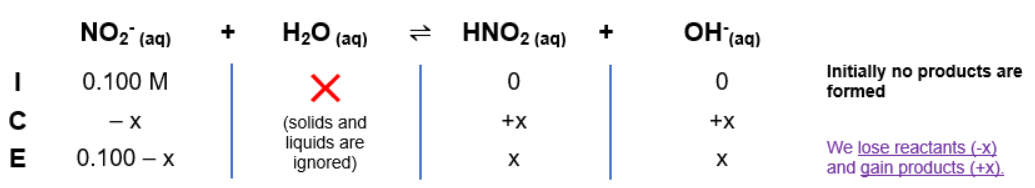# Problem: Knowing that NaNO2 is the salt of a strong base and weak acid calculate : a: pH of 0.100 M NaNO2 solution. Ka of HNO2 = 4.6 x 10-4.

89% (67 ratings)
###### FREE Expert Solution

To calculate pH of starting NaNO2 solution and after each addition of HCl

a) NaNO2 dissociates into ions: Na+ + NO2- and reacts with water:

• NO2- is conj. base of weak acid HNO2

Step 1: ICE ChartThe Kb expression for NO2-  is:

$\overline{){{\mathbf{K}}}_{{\mathbf{b}}}{\mathbf{=}}\frac{\mathbf{products}}{\mathbf{reactants}}{\mathbf{=}}\frac{\mathbf{\left[}{\mathbf{HNO}}_{\mathbf{2}}\mathbf{\right]}\mathbf{\left[}{\mathbf{OH}}^{\mathbf{-}}\mathbf{\right]}}{\mathbf{\left[}{\mathbf{NO}}_{\mathbf{2}}\mathbf{-}\mathbf{\right]}}}$

Calculate Kb:

89% (67 ratings)###### Problem Details

Knowing that NaNO2 is the salt of a strong base and weak acid calculate :

a: pH of 0.100 M NaNO2 solution. Ka of HNO2 = 4.6 x 10-4.